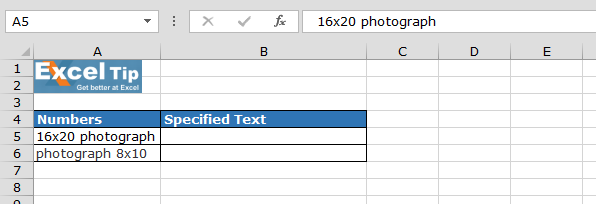# If a Cell Contains a Certain Text Then Put a Specified Number in another Cell

In this article we will learn how to specified number, if a cell contains certain text. We will use the combination of IF, ISNUMBER and SEARCH functions in Microsoft Excel.

Let’s understand with a simple exercise how we can specify the text in a cell based on the text in another cell. We have 2 numbers in the range A2:A4. Now we want to specify the text in range B2:B3.To specify the text based on number follow the below mentioned steps:-

• Select the cell B5, and write the formula in the cell
• =IF(ISNUMBER(SEARCH("16x20",A5)), "Baseball,BaseballPhotos16x20", IF(ISNUMBER(SEARCH("8x10",A5)),"Baseball,Basebal lPhotos8x10",""))
• Press Enter on the keyboard
• The function will return “Baseball,BaseballPhotos16x20”
• Paste the same formula in range B6

SEARCH function will search the number in cell, if the searched number will be in cell then function will return numeric value else #VALUE! Error.

On the basis of Search function’s result ISNUMBER function will give the result in TRUE or FALSE.

IF function will check the logical test is true then result is set, in condition of FALSE will check for another value.

This is the method you can follow if you want to put specified text with a number in one cell based on the data in another cell.We would love to hear from you, do let us know how we can improve, complement or innovate our work and make it better for you. Write us at info@exceltip.com

## Users are saying about us...

1.I have address fields that contain the street address, city and ZIP code and, of course, the strings are of different lengths; I need to be able to either parse strings of variable lengths, or search each field for a particular city (i.e. West Palm Beach) and enter a result in another cell. The first option would be the most ideal - is there a way to achieve this goal? Thank you.

2.Hi Steve Smith,

Can you send us your Excel file or mention here your question in Excel format.

Thanks

3.This Formula =IF(ISNUMBER(SEARCH(“16×20?,A2)), “Baseball,BaseballPhotos16x20?, IF(ISNUMBER(SEARCH(“8×10?,A2)),”Baseball,Basebal lPhotos8x10?,””))

If We Want To Place A Different Value in Place of False, What Should i Do??

4.What if I have more than 150 such cases where I have to use IF statement ? Excel is not allowing m eto add more than 64 if statements in a single formula.

5.I need help with a similar formula,

So what I have is loads of data, I then need to rate each one in a class between 1 - 9. Each class has a value range.
Example:
85
72
80
64
88

Class 1 = 96

6.My customer has a program where they pick what items they need to order and then they send it to me to requesting pricing for the list of items. The description of the items are always the same. But they only send what items they need for that week.

The list is always in one column with 1 item per row.

Is there a way that I can search a column and have the formula give the price in the next column / same row?

Ex: If in column c they ask for 2" conduit (let's say it happens to be in row 24 today). How can I get it search the column and automatically put the price of \$2.12 in the next column ( column d, row 24)?

7.